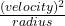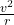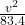Question

A car of mass 410 kg travels around a flat, circular race track of radius 83.4 m. The coefficient of static friction between the wheels and the track is 0.286. The acceleration of gravity is 9.8 m/s 2 . What is the maximum speed v that the car can go without flying off the track

1.The maximum speed v that the car can go without flying off the track = 15.29 m/s

Explanation:

let us first lay out the information clearly:

mass of car (m) = 410 kg

radius of race track (r) = 83.4 m

coefficient of friction (μ) = 0.286

acceleration due to gravity (g) = 9.8 m/s²

maximum speed = v m/s

For a body in a constant circular motion, the centripetal for (F) acting on the body is given by:

F = mass × ω

where:

F = maximum centripetal force = mass × μ × g

ω = angular acceleration =∴ F = mass × ω

m × μ × g = m ×410 × 0.286 × 9.8 = 410 ×since 410 is on both sides, they will cancel out:

0.286 × 9.8 =2.8028 =now, we cross-multiply the equation

2.8028 × 83.4 == 233.754

∴ v = √(233.754)

v = 15.29 m/s

Therefore, the maximum speed v that the car can go without flying off the track = 15.29 m/s